# Designing Finite Automata from Regular Expression (Set 5)

• Difficulty Level : Hard
• Last Updated : 18 Jan, 2022

In the below article, we shall see some Designing of Non-deterministic Finite Automata form the given Regular Expression-

As NFA can be changed to corresponding DFA.

Regular Expression 1: Regular language,

`L1 = ((a+b)(a+b)(a+b))* `

The language of the given RE is,

`{aaa, aba, baa, bba, aab, abb, bab, bbb,...} `

Length of the string is divisible by 3 ((length of string) mod 3 = 0).
Its finite automata will be like below-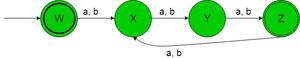In the above transition diagram, as we can see that initial state ‘W’ on getting either ‘a’ or ‘b’ as the input it transits to a state ‘X’ and so on for the remaining states. Thus this FA accepting all the strings of the given RE language.

Regular Expression 2: Regular language,

`L2 = (a+b)(a+b).((a+b)(a+b)(a+b))* `

The language of the given RE is,

`{aa, ab, ba, bb, aaaaa, aabab, ..........} `

Length of string mod 3 = 2
Its finite automata will be like below-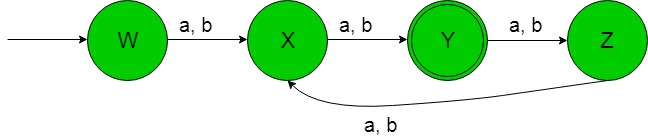In the above transition diagram, as we can see that initial state ‘W’ on getting either ‘a’ or ‘b’ as the input it transits to a state ‘X’ and so on for the remaining states. Thus this FA accepting all the strings of the given RE language.

Regular Expression 3: Regular language,

`L3 = b*ab*ab* `

The language of the given RE is,

`{baa, babab, bbabbabb, ......} `

Number of ‘a’ exactly 2.
Its finite automata will be like below-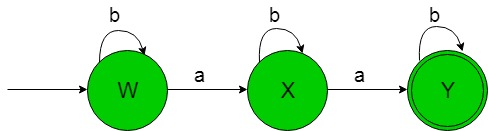In the above transition diagram, as we can see that initial state ‘W’ on getting ‘b’ as the input it remains in the state of itself and on getting ‘a’ as the input it transits to a state ‘X’ so on for the remaining states. Thus this FA accepting all the strings of the given RE language.

Regular Expression 4: Regular language,

`L4 = b*ab*a(a+b)* `

The language of the given RE is,

`{baa, babab, bbabbaabb, ......} `

Number of ‘a’ atleast 2.
Its finite automata will be like below-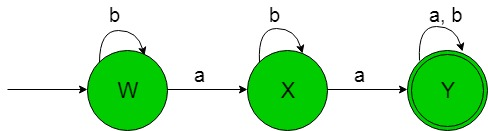In the above transition diagram, as we can see that initial state ‘W’ on getting ‘b’ as the input it remains in the state of itself and on getting ‘a’ as the input it transits to a state ‘X’ so on for the remaining states. Thus this FA accepting all the strings of the given RE language.

Regular Expression 5: Regular language,

`L5 = b*(ε+a)b*(ε+a)b* `

The language of the given RE is,

`{b, bb, bbb, bab, baab, babab, bbab, .....} `

Number of ‘a’ at most 2.
Its finite automata will be like below-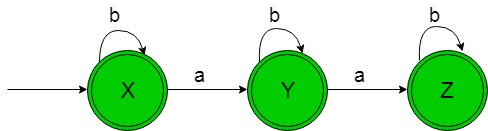In the above transition diagram, as we can see that initial and final state ‘X’ on getting ‘b’ as the input it remains in the state of itself and on getting ‘a’ as the input it transits to another final state ‘Y’ and so on for the remaining states. Thus this FA accepting all the strings of the given RE language.

My Personal Notes arrow_drop_up
Recommended Articles
Page :